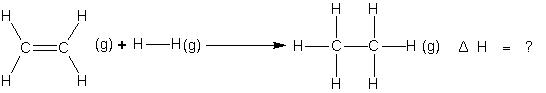Click to Chat

1800-1023-196

+91-120-4616500

CART 0

• 0

MY CART (5)

Use Coupon: CART20 and get 20% off on all online Study Material

ITEM
DETAILS
MRP
DISCOUNT
FINAL PRICE
Total Price: Rs.

There are no items in this cart.
Continue Shopping• Complete Physics Course - Class 11
• OFFERED PRICE: Rs. 2,968
• View Details

```Application of bond energies

(i) Determination of enthalpies of reactions

Suppose we want to determine the enthalpy of the reaction.If bond energies given for C ¾ C, C = C, C¾H, and H ¾ H are 347.3, 615.0, 416.2 and 435.1KJ mol-1 respectively.

ΔH = ΔHC=C + ΔHH–H + 4ΔHC–H – (ΔHC–C + 6ΔHC–H)

= (615.0 + 435.1) – (347.3 + 832.4) => –129.6 KJ

(ii) Determination of enthalpies of formation of compounds

Consider the formation of acetone.

H     O     H

|       ||      |

3C(g) + 6H(g) + O(g) ———> H — C — C — C — H          ΔH = ?

|               |

H              H

ΔHf = [3ΔHH–H + 1/2 ΔH0–0 + 3ΔHC(s)—>C(g)] – [2ΔHC–C + 6ΔHC–H + ΔHC=O]

by putting the value of different bond energies you can determine the  ΔHf .

(iii) Determination of resonance energy

If a compound exhibits resonance, there is a considerable difference between the enthalpies of formation as calculated from bond energies and those determined experimentally. As an example we may consider the dissociation of benzene.

C6H6 (g) ———> 6C(g) + 6H(g)

Assuming that benzene ring consists of three single and three double bonds (Kekule’s structure) the calculated dissociation energy comes out to be 5384.1 KJ from bond energies data.

ΔHd = 3ΔHC–C + 3ΔHC=C + 6ΔHC–H

The experimental value is known to be 5535.1 KJ/mol. Evidently, the energy required for the dissociation of benzene is 151 KJ more that the calculated value. The difference of 151 KJ gives the resonance energy of benzene.

Exercise:

Calculate the enthapy of combustion of benzene (l) on the basis of the following.

(i) Resonance energy of benzene (l) = – 152 kJ mole–1

(ii) Enthalpy of hydrogenation of cyclohexene (l) = – 119 kJ mole–1

(iii) (ΔHf0)C6H12 = – 156 kJ mole–1

(iv) (ΔH0f)H2O = – 285.8 kJ mole–1

(v) (ΔHf0)CO2 = – 393.5 kJ mole–1

To read more, Buy study materials of Thermodynamics comprising study notes, revision notes, video lectures, previous year solved questions etc. Also browse for more study materials on Chemistry here.
```### Course Features

• 101 Video Lectures
• Revision Notes
• Previous Year Papers
• Mind Map
• Study Planner
• NCERT Solutions
• Discussion Forum
• Test paper with Video Solution